Courses

# Shear Stresses in Beams Notes | EduRev

## Mechanical Engineering : Shear Stresses in Beams Notes | EduRev

The document Shear Stresses in Beams Notes | EduRev is a part of the Mechanical Engineering Course Strength of Materials (SOM).
All you need of Mechanical Engineering at this link: Mechanical Engineering

Shearing Stress

In the theory of bending, presence of shear and the distortion of plane sections was neglected because the effect of it is not on bending stress is not of practical importance.

• But, it is important to consider the shear stresses for their own importance.
• Here, only the shearing stresses in the transverse palnes parallel to the shearing force and the complimentary shear stresses in the longitudinal planes parallel to the axis of the beam.
Shear stress distribution over rectangular section
F​ig. shows the rectangular cross-section of the beam, over which we have to determine the distribution of shear stress. Consider a layer ab at any above the N.A.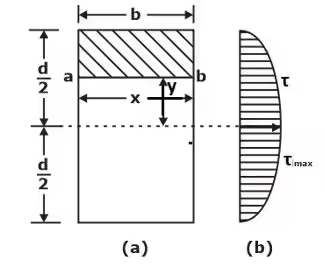Shearing stress on a layer JK of beam at distance y from neutral axis.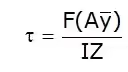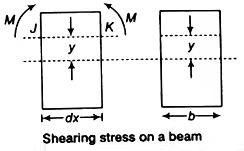Where,

• V = Shearing force
•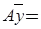First moment of area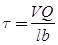Shear stress in Rectangular Beam
• Suppose, we have to determine the shear stress at the longitudinal layer having y distance from neutral axis.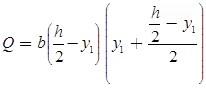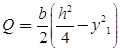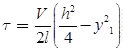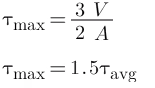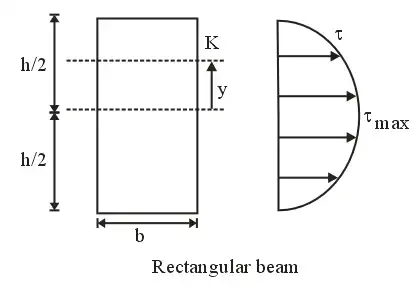Circular Beam
• Centre of gravity of semi-circle lies at distance from centre or base line. As it is symmetrical above neutral axis, hence at neutral axis shear stress will be maximum.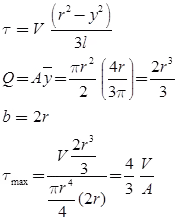• For τmax substituting y = 0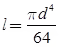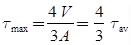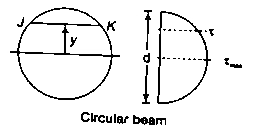Shears Stress in Hollow Circular Cross-Section

• In hollow circular cross-section, if we have to calculate τ at neutral axis by the formula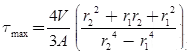Shear Stress in Triangular Section

• In a triangular cross-section, if we have to calculate τ at neutral axis, then in formula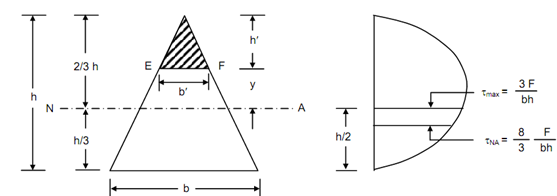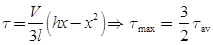Shear Stress in I-section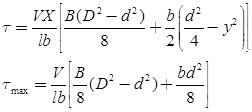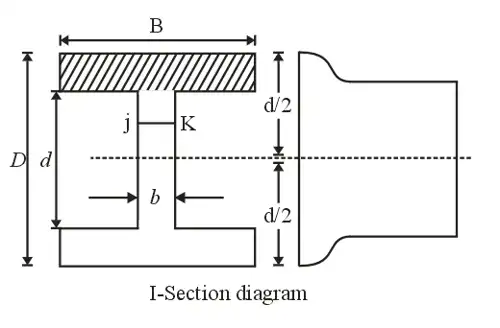Combined Stresses

Under combination of

• Direct Stress
σd = P/A

where P = axial thrust, A = area of cross-section

• Bending Stress
σb = My/l

where M = bending moment, y- distance of fibre from neutral axis, I = moment of inertia.

• Shear stresses
Τ = Tr/J

where T = torque, r = radius of shaft, J = polar moment of inertia.

Combined Stress is:

• Equivalent Torsional Moment:-
The equivalent torsional moment is defined as the torsional moment, which when acting alone, will produce the same torsional shear stress in the shaft as under the combined action of bending moment (Mb) and torsional moment (Mt)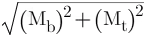• Equivalent Bending Moment:-
The equivalent Bending moment is defined as the bending moment, which when acting alone, will produce the same bending stresses (tensile and compressive) in the shaft as under the combined action of bending moment (Mb) and torsional moment (Mt)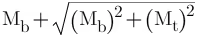Example:- A shaft of diameter 8 cm is subjected to a bending moment of 3000 Nm and a twisting moment of 4000 Nm. The maximum normal stress induced in the shaft is equal to
Solution:-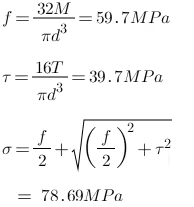• Stress due to combined direct and bending load:
Suppose a beam under direct compressive and bending load as shown in the diagram. where A = Area,
y = distance of extreme fiber from Neutral axis and I = moment of Inertia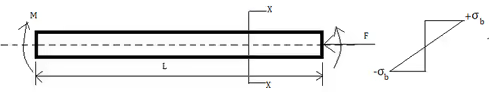Direct compressive stress σ = F/A
Bending stress will vary linearly from center to extreme fiber
σb = My/I
so, total stress at upper fibre = Direct compressive stress+ tensile stress due to a bending load
= (-σ)+σb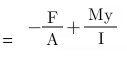Same for lower extreme fiber = Direct compressive stress+ Compressive stress due to bending load
=(-σ)+(-σb)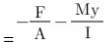Example-
For the component loaded with a force F as shown in the figure, the axial stress at the corner point P is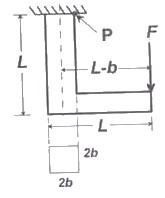Solution:-
At point P two types of stress are acting, bending & axial tensile load

So, bending stress =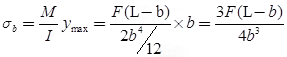Axial tensile stress =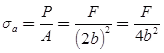Total axial stress at P = σb + σa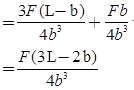Offer running on EduRev: Apply code STAYHOME200 to get INR 200 off on our premium plan EduRev Infinity!

## Strength of Materials (SOM)

35 videos|33 docs|48 tests

,

,

,

,

,

,

,

,

,

,

,

,

,

,

,

,

,

,

,

,

,

;